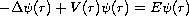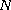Electron. J. Diff. Eqns., Vol. 2007(2007), No. 129, pp. 1-11.

### Spectral bisection algorithm for solving Schrodinger equation using upper and lower solutions Qutaibeh Deeb Katatbeh

Abstract:
This paper establishes a new criteria for obtaining a sequence of upper and lower bounds for the ground state eigenvalue of Schrodinger equationinspatial dimensions. Based on this proposed criteria, we prove a new comparison theorem in quantum mechanics for the ground state eigenfunctions of Schrodinger equation. We determine also lower and upper solutions for the exact wave function of the ground state eigenfunctions using the computed upper and lower bounds for the eigenvalues obtained by variational methods. In other words, by using this criteria, we prove that the substitution of the lower(upper) bound of the eigenvalue in Schrodinger equation leads to an upper(lower) solution. Finally, two proposed iteration approaches lead to an exact convergent sequence of solutions. The first one uses Raielgh-Ritz theorem. Meanwhile, the second approach uses a new numerical spectral bisection technique. We apply our results for a wide class of potentials in quantum mechanics such as sum of power-law potentials in quantum mechanics.

Submitted July 18, 2007. Published October 4, 2007.
Math Subject Classifications: 34L16, 81Q10.
Key Words: Schrodinger equation; lower solution; upper solution; spectral bounds; envelope method.

Show me the PDF file (257 KB), TEX file, and other files for this article.Qutaibeh Deeb Katatbeh Department of Mathematics and Statistics Jordan University of Science and Technology Irbid, Jordan 22110 email: qutaibeh@yahoo.com